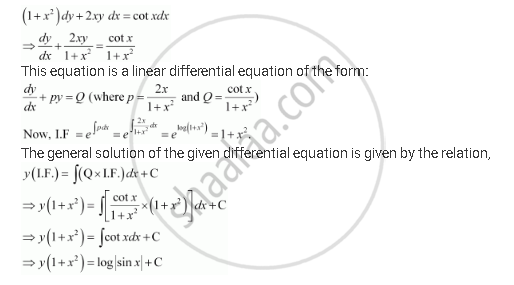Share

# For the Differential Equations Find the General Solution: (1 + X2) Dy + 2xy Dx = Cot X Dx (X ≠ 0) - CBSE (Commerce) Class 12 - Mathematics

ConceptMethods of Solving First Order, First Degree Differential Equations Linear Differential Equations

#### Question

For the differential equations find the general solution: (1 + x2) dy + 2xy dx = cot x dx (x ≠ 0)

#### SolutionIs there an error in this question or solution?

#### APPEARS IN

NCERT Solution for Mathematics Textbook for Class 12 (2018 to Current)
Chapter 9: Differential Equations
Q: 8 | Page no. 413

#### Video TutorialsVIEW ALL 

Solution For the Differential Equations Find the General Solution: (1 + X2) Dy + 2xy Dx = Cot X Dx (X ≠ 0) Concept: Methods of Solving First Order, First Degree Differential Equations - Linear Differential Equations.
S